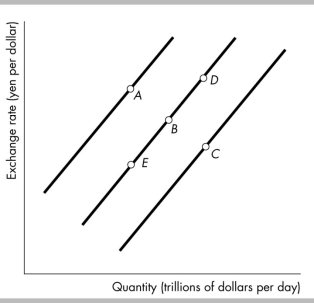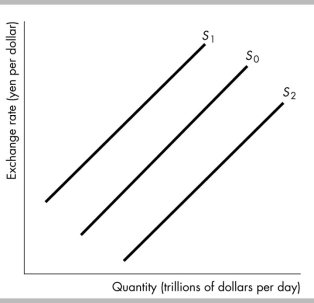• FAQ
• Contact/
/
/
51) The supply of dollars in the foreign exchange market
Not my Question
Flag Content

# Question : 51) The supply of dollars in the foreign exchange market : 1910687

51) The supply of dollars in the foreign exchange market decreases and that the supply curve shifts leftward if

A) the U.S. interest rate differential decreases.

B) the expected future exchange rate rises.

C) the exchange rate for the dollar rises.

D) All of the above are correct.52) In the figure above, suppose the economy is initially at point B. Then the interest rate in Japan rises relative to the interest rate in the United States. This change ________ the supply of dollars and the market moves to a point such as ________.

A) decreases; A

B) decreases; E

C) increases; D

D) increases; C

53) In the figure above, suppose the economy is initially at point B. If people come to believe that the exchange rate will fall in the future, the supply of dollars ________ and the market moves to point such as ________.

A) decreases; A

B) decreases; E

C) increases; D

D) increases; C54) In the figure above, the shift in the supply curve for U.S. dollars from S0 to S1 could occur when

A) the U.S. interest rate differential increases.

B) the U.S. interest rate differential decreases.

C) the expected future exchange rate falls.

D) the current exchange rate falls.

55) In the figure above, the shift in the supply curve for U.S. dollars from S0 to S1 could occur when

A) the U.S. interest rate falls.

B) foreign interest rates fall.

C) the expected future exchange rate falls.

D) the current exchange rate falls.

56) In the figure above, the shift in the supply curve for U.S. dollars from S0 to S1 could occur when

A) the U.S. interest rate rises.

B) foreign interest rates rise.

C) the expected future exchange rate falls.

D) the current exchange rate rises.

57) In the figure above, the shift in the supply curve for U.S. dollars from S0 to S2 could occur when

A) the U.S. interest rate falls.

B) the expected future exchange rate rises.

C) the U.S. interest rate differential increases.

D) the current exchange rate falls.

58) In the figure above, the shift in the supply curve for U.S. dollars from S0 to S2 could occur when

A) foreign interest rates rise.

B) the expected future exchange rate rises.

C) the U.S. interest rate rises.

D) the current exchange rate falls.

59) In the figure above, the shift in the supply curve for U.S. dollars from S0 to S1 could occur when

A) the expected future exchange rate rises.

B) the U.S. interest rate differential decreases.

C) the expected future exchange rate falls.

D) the current exchange rate falls.

60) In the figure above, the shift in the supply curve for U.S. dollars from S0 to S2 could occur when

A) the current exchange rate rises.

B) the current exchange rate falls.

C) the expected future exchange rate rises.

D) the expected future exchange rate falls.

## Solution 5 (1 Ratings )

Solved
Marketing 7 Months Ago 15 Views